Hiker and cyclist

At 7 PM hiker started at 6 km/h to hike. After 3 hrs. later started on the same trip cyclist at speed of 15 km/h. After an hour of driving a flat tire and take him half hours to correct defect. At what time catch up cyclist with hiker?

Result

t = 12:50 hSolution:

6(t-7.00) = 15(t-(7.00+3.00+0.5))

9t = 115.5

t = 776 ≈ 12.833333

Calculated by our simple equation calculator.

Leave us a comment of this math problem and its solution (i.e. if it is still somewhat unclear...):Be the first to comment!To solve this verbal math problem are needed these knowledge from mathematics:

Do you have a linear equation or system of equations and looking for its solution? Or do you have quadratic equation? Do you want to convert length units?

Next similar math problems:

1. Pedestrian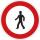Pedestrian came started at 8h in the morning with speed 4.4 km/h. At half-past eleven cyclist started at 26 km/h same way. How many minutes take cyclist to catch up pedestrian?
2. Warehouse carsFrom the warehouse started truck at speed 40km/h. After 1hour 30mins started from the same place same direction a car at speed 70 km/h. For how long and at what distance from the warehouse overtake a truck?
3. Two citiesCities A and B are 200 km away. At 7 o'clock from city A, the car started at an average speed of 80 km/h, and from B at 45 min later the motorcycle is started at an average speed of 120 km/h. How long will they meet and at what distance from the point A it
4. Steamer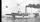At 6 hours 40 minutes steamer sailed from the port at speed 12 km/h. At exactly 10 hours started sail motorboat at speed 42 km/h. When motorboat will catch steamer?
5. Two trains meetFrom A started at 7:15 express train at speed 85 km/h to B. From B started passenger train at 8:30 in the direction to A and at speed 55 km/h. The distance A and B is 386 1/4 km. At what time and at what distance from B the two trains meet?
6. Holiday SMS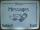Kucera and Malek start from the holiday together. They agreed that if they be 100 km apart, will send a SMS. Kucera traveling at 60 km/h., Malek 90 km/h. They started at 14 hours PM. At what time they send a message?
7. Theorem proveWe want to prove the sentence: If the natural number n is divisible by six, then n is divisible by three. From what assumption we started?
8. Otto and Joachim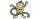Otto and Joachim go through the woods. After some time Otto tire and make 15 minutes stop. Joachim meanwhile continues at 5 km/h. Otto when he set off again, first running speed of 7 km/h, but it keep only 30 sec and 1 minute must continue at 3 km/h. This.
9. Moving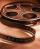Vojta left the house at three o'clockat 4 km/h. After half hour later went from the same place Filip by bicycle at speed 18 km/h. How long take Tilip to catch up Vojta and how far from the house?
10. FamilyMom is 42 years old and her daughters 13 and 19. After how many years will mother as old as her daughter together?
11. Skier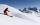At this point, the first skier lead 20 km before the second skier and travels at a constant speed 19 km/h. The second skier rides at 24 km/h. How long take him to catch up the first?
12. StormSo far, a storm has traveled 35 miles in 1/2 hour in direction straight to observer. If it is currently 5:00 p. M. And the storm is 105 miles away from you, at what time will the storm reach you? Explain how you solved the problem.
13. WavelengthCalculate the wavelength of the tone frequency 11 kHz if the sound travels at speeds of 343 m/s.
14. Mom and daughterMother is 39 years old. Her daughter is 15 years. For many years will mother be four times older than the daughter?
15. Six yearsIn six years Jan will be twice as old as he was six years ago. How old is he?
16. Walnutsx walnuts were in the mission. Dano took 1/4 of nuts Michael took 1/8 from the rest and John took 34 nuts. It stayed here 29 nuts. Determine the original number of nuts.
17. Fifth of the numberThe fifth of the number is by 24 less than that number. What is the number?